Sequences Informally a sequence is a set of

• Slides: 15Sequences • Informally, a sequence is a set of elements written in a row. – This concept is represented in CS using onedimensional arrays • The goal of mathematics in general is to identify, prove, and utilize patterns – The most common way to do this is to use sequences to represent outputs – The most efficient way to use sequences is through computer science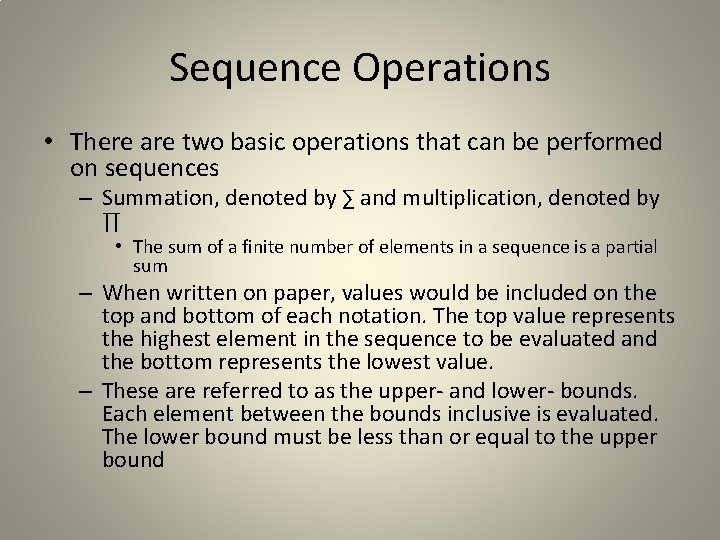Sequence Operations • There are two basic operations that can be performed on sequences – Summation, denoted by ∑ and multiplication, denoted by ∏ • The sum of a finite number of elements in a sequence is a partial sum – When written on paper, values would be included on the top and bottom of each notation. The top value represents the highest element in the sequence to be evaluated and the bottom represents the lowest value. – These are referred to as the upper- and lower- bounds. Each element between the bounds inclusive is evaluated. The lower bound must be less than or equal to the upper bound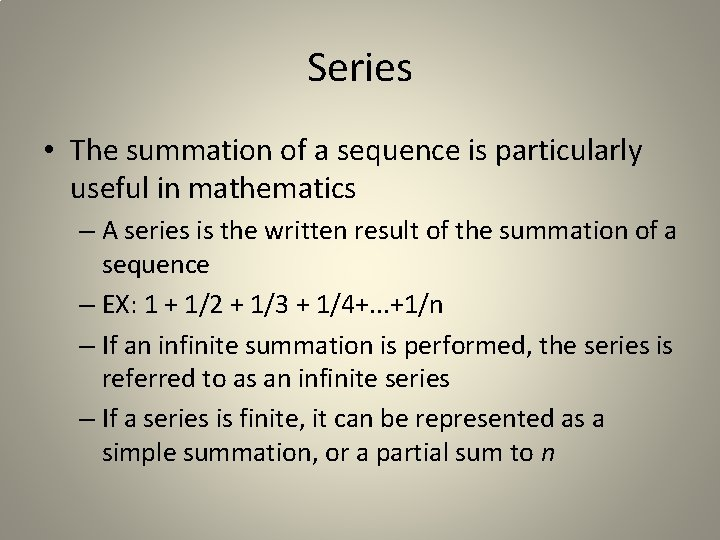Series • The summation of a sequence is particularly useful in mathematics – A series is the written result of the summation of a sequence – EX: 1 + 1/2 + 1/3 + 1/4+. . . +1/n – If an infinite summation is performed, the series is referred to as an infinite series – If a series is finite, it can be represented as a simple summation, or a partial sum to nExamples • Summation: – If n = 3, the summation of 2 raised to k is 2^1 + 2^2 + 2^3 = 14 – The general summation formula is • Products: – If n = 3, m = 1 and the formula is (k+1) then the answer is (1+1)(2+1)(3+1) = 2*3*4 = 24Recursive Form of Summation • m and n are any integers, n > m – – This definition lets you separate out the final term or condense a complex statement into a smaller one. – EX: a telescoping sum • By breaking the term into two parts, you cancel and simplify the summation formula • • Everything from 1 to 1/(n+1) cancels out, so the final formula for the sum isRecursive Form of Products • m and n are any integers, n > m – A common use of recursive product form is computing the factorial, n!, which is the product of n and each integer less than n and greater than 1 inclusive. Note that 0! = 1 – The recursive definition of n! is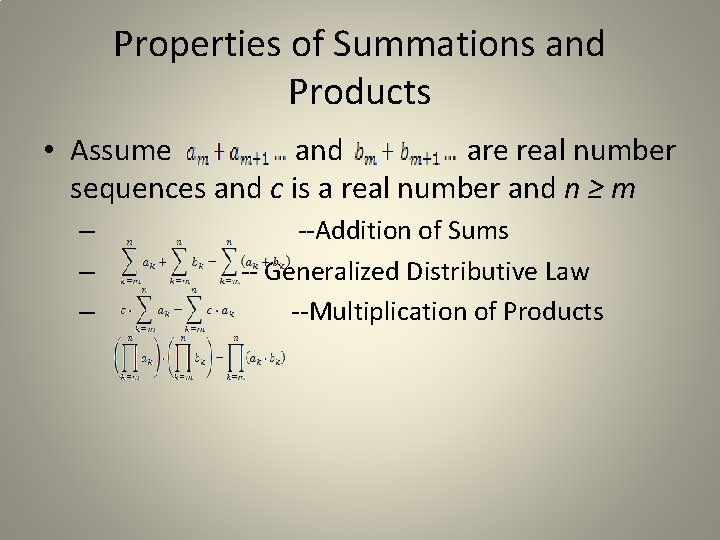Properties of Summations and Products • Assume and are real number sequences and c is a real number and n ≥ m – – – --Addition of Sums -- Generalized Distributive Law --Multiplication of Products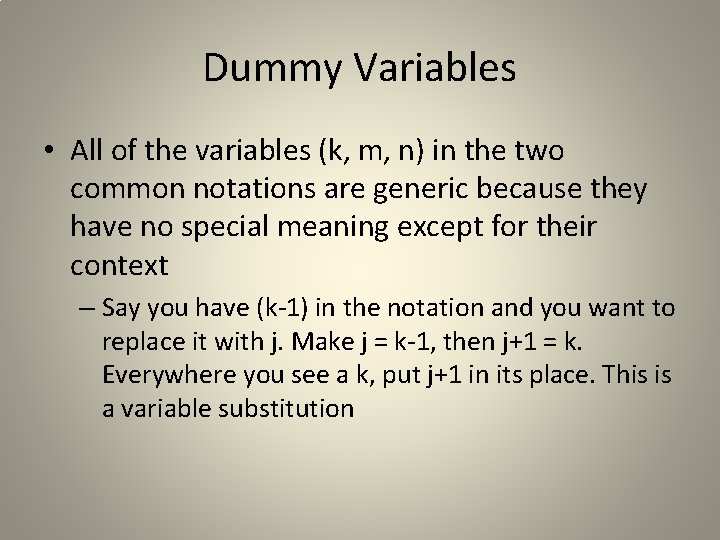Dummy Variables • All of the variables (k, m, n) in the two common notations are generic because they have no special meaning except for their context – Say you have (k-1) in the notation and you want to replace it with j. Make j = k-1, then j+1 = k. Everywhere you see a k, put j+1 in its place. This is a variable substitution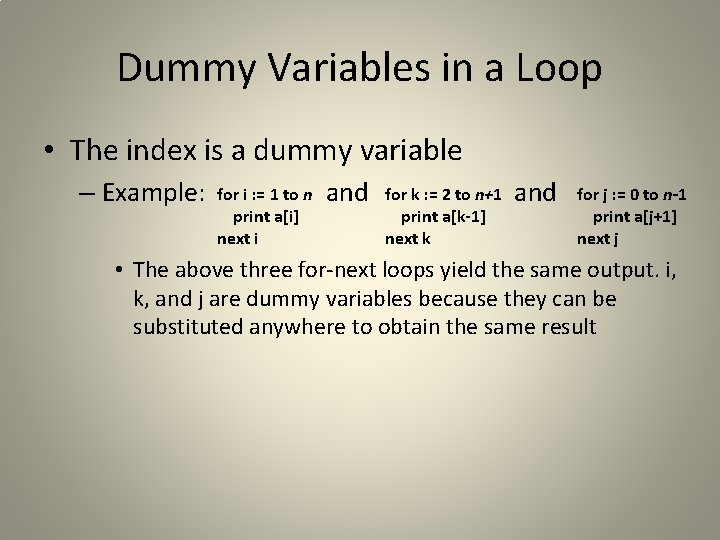Dummy Variables in a Loop • The index is a dummy variable – Example: for i : = 1 to n print a[i] next i and for k : = 2 to n+1 print a[k-1] next k and for j : = 0 to n-1 print a[j+1] next j • The above three for-next loops yield the same output. i, k, and j are dummy variables because they can be substituted anywhere to obtain the same result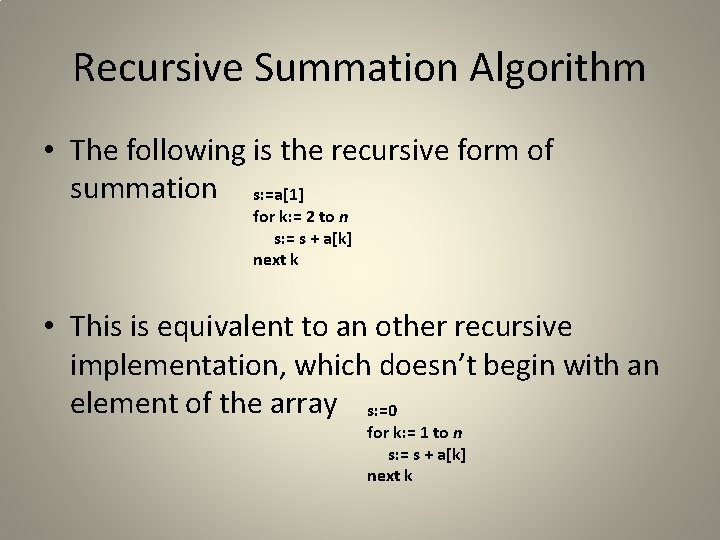Recursive Summation Algorithm • The following is the recursive form of summation s: =a for k: = 2 to n s: = s + a[k] next k • This is equivalent to an other recursive implementation, which doesn’t begin with an element of the array s: =0 for k: = 1 to n s: = s + a[k] next k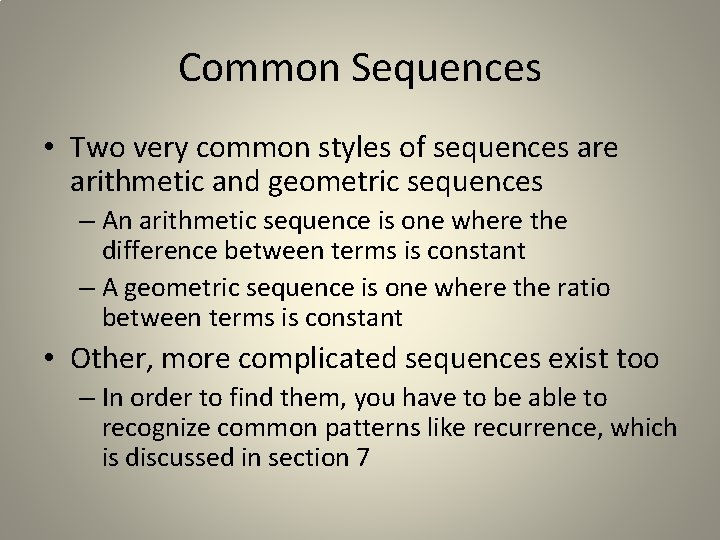Common Sequences • Two very common styles of sequences are arithmetic and geometric sequences – An arithmetic sequence is one where the difference between terms is constant – A geometric sequence is one where the ratio between terms is constant • Other, more complicated sequences exist too – In order to find them, you have to be able to recognize common patterns like recurrence, which is discussed in section 7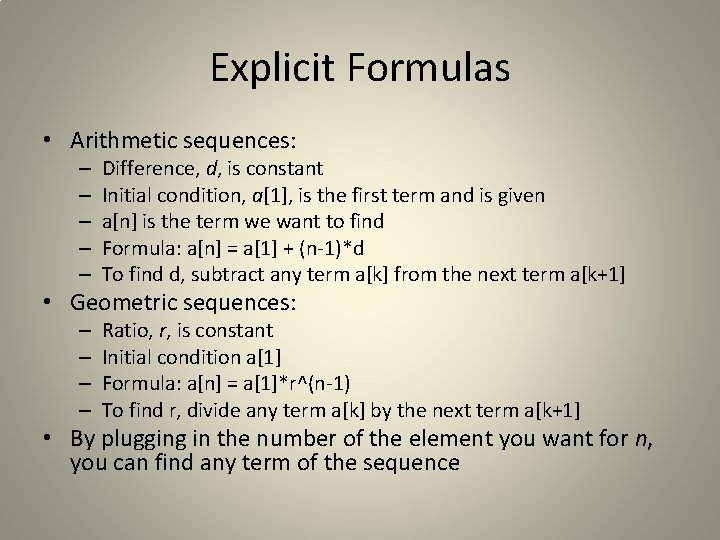Explicit Formulas • Arithmetic sequences: – – – Difference, d, is constant Initial condition, a, is the first term and is given a[n] is the term we want to find Formula: a[n] = a + (n-1)*d To find d, subtract any term a[k] from the next term a[k+1] • Geometric sequences: – – Ratio, r, is constant Initial condition a Formula: a[n] = a*r^(n-1) To find r, divide any term a[k] by the next term a[k+1] • By plugging in the number of the element you want for n, you can find any term of the sequenceSigma Notation for a Series • A sigma, as earlier denoted, means summation – Sigma notation represents the series up to a certain element n – The sigma notation is equivalent to the summation formula for a sequence – Two formulas to know for now are the summations of arithmetic and geometric sequences – for arithmetic for geometric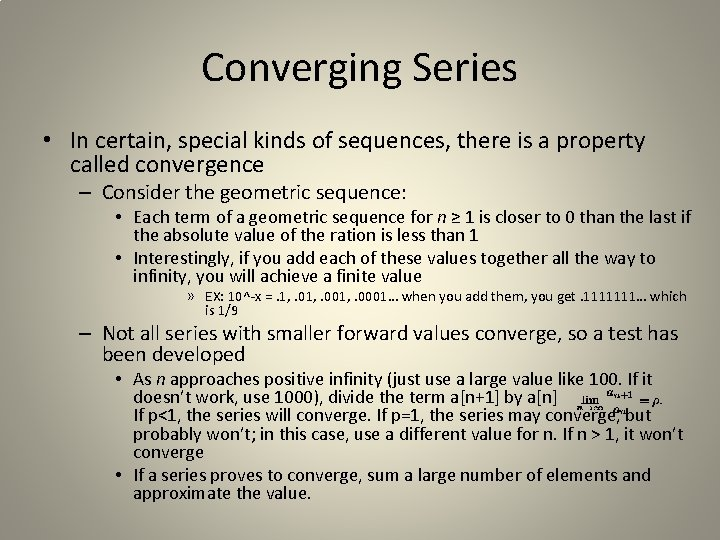Converging Series • In certain, special kinds of sequences, there is a property called convergence – Consider the geometric sequence: • Each term of a geometric sequence for n ≥ 1 is closer to 0 than the last if the absolute value of the ration is less than 1 • Interestingly, if you add each of these values together all the way to infinity, you will achieve a finite value » EX: 10^-x =. 1, . 001, . 0001… when you add them, you get. 1111111… which is 1/9 – Not all series with smaller forward values converge, so a test has been developed • As n approaches positive infinity (just use a large value like 100. If it doesn’t work, use 1000), divide the term a[n+1] by a[n] If p<1, the series will converge. If p=1, the series may converge, but probably won’t; in this case, use a different value for n. If n > 1, it won’t converge • If a series proves to converge, sum a large number of elements and approximate the value.Finding Infinite Sums • Once a series is proven to converge, an effort can be made to find its infinite sum – To calculate the exact value of an infinite sum requires mathematical analysis, such as calculus, in most situations – For this level of discrete mathematics, approximations of infinite sums will do just fine – To approximate, use a for loop to add successive terms (up to a high number, like 10, 000)